# Electronics and Communication Engineering - Automatic Control Systems

### Exercise :: Automatic Control Systems - Section 1

46.

The system in the given figure, has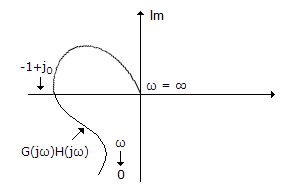A. good gain and phase margin B. poor gain and phase margin C. good gain margin but poor phase margin D. poor gain margin but good phase margin

Explanation:

Since the plot is very near (-1 + j0) point, gain margin is poor.

47.

The given figure shows a pole zero diagram. The transfer function G(j1) is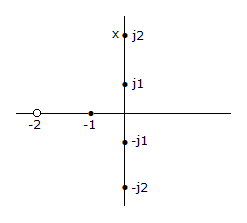A. 0.5 ∠0 B. 2.7 ∠- 31° C. 2 ∠45° D. 2 ∠- 67.4°

Explanation:

Join j1 point with the zero and poles. Find magnitude and phase angles of all these lines.

48.

For very low frequencies, v0/vi in the given figure equals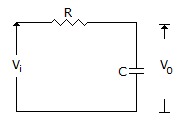A. 1 B. 0 C. ∞ D. 0.5

Explanation:

For low frequencies Xc is very high as compared to R. Hence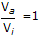.

49.

In a minimum phase system

 A. all poles lie in the left half plane B. all zeros lie in the left half plane C. all poles lie in the right half plane D. all except one pole or zero lie in the left half plane

Explanation:

For stability poles must be in left half plane. Zeros in left half plane lead to minimum phase shift.

50.

For the system of the given figure the transfer function is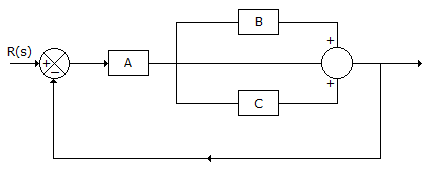A.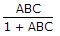B.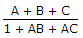C.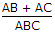D.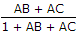Transfer function =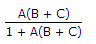.